# 3.2. 开发板 - Pika 派 Zero

## 3.2.1. 开发板获取

https://item.taobao.com/item.htm?spm=a21dvs.23580594.0.0.52de3d0dt7rqAx&ft=t&id=654947372034

• Lite 青春版：STM32G030 + CH340 串口芯片 64k flash 8k ram

• Pro 专业版：STM32G030 + DAPLink 调试器 64K flash 8k ram

• Plus 顶配版：STM32G070 + DAPLink 调试器 128k flash 32k ram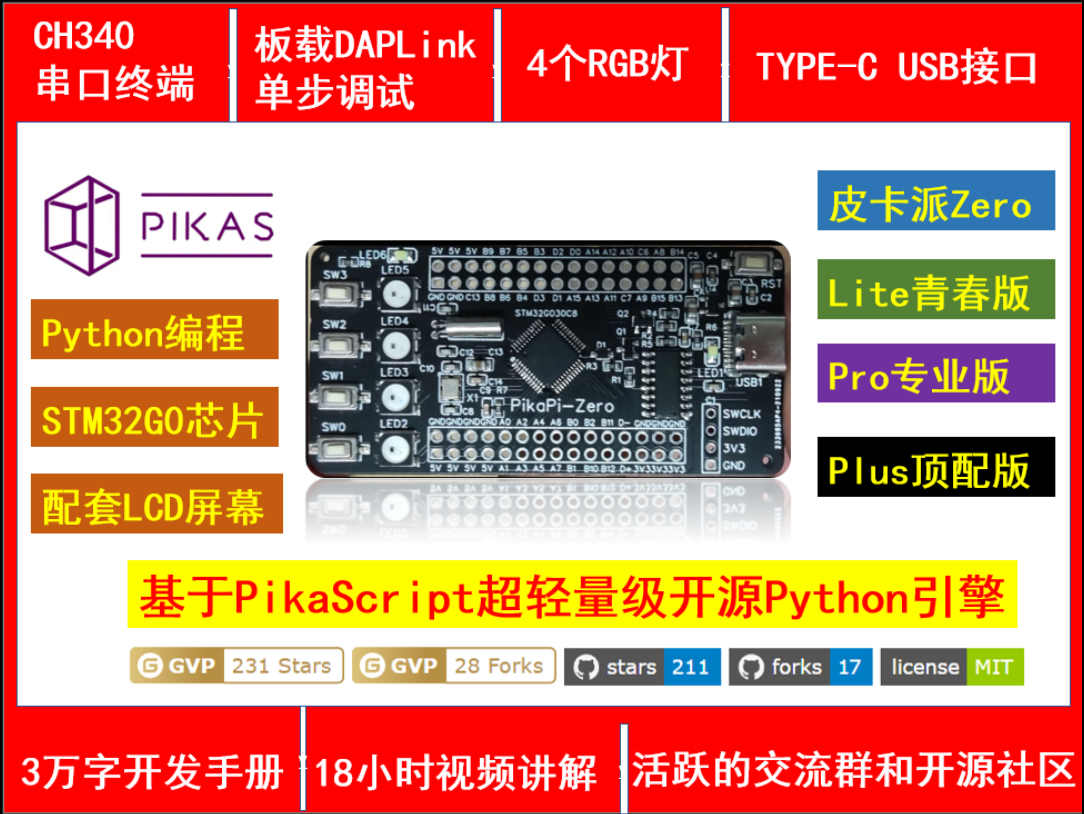## 3.2.2. 视频教程

https://space.bilibili.com/5365336/channel/seriesdetail?sid=1034902

## 3.2.3. 固件升级

【重要】！ 拿到开发板之后，先升级固件，否则不能运行例程！

### 3.2.3.1. 编译固件

Lite 版和 Pro版使用 stm32g030 平台。

Plus 版使用 stm32g070 平台。

（选择平台后会自动选择默认的模块)[注意]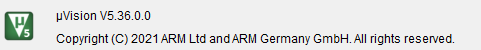### 3.2.3.2. 使用SWD升级

Lite 版和 Pro 版使用 bsp/stm32g030 工程。

Plus 版使用 bsp/stm32g070 工程。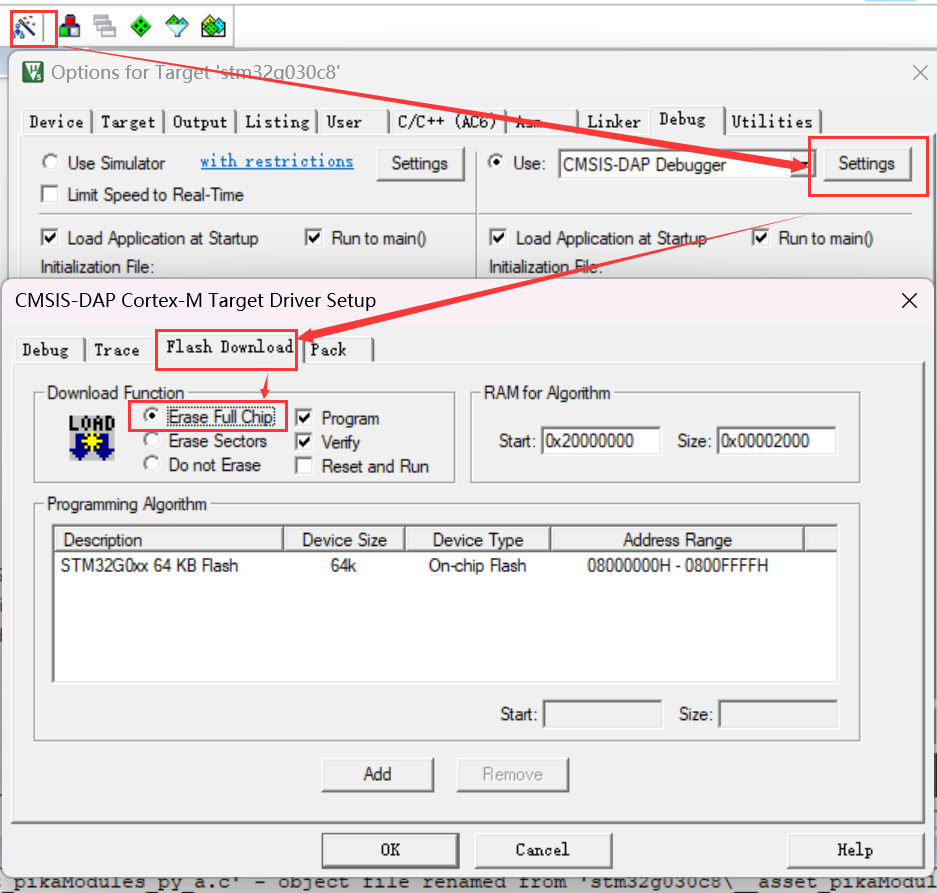## 3.2.4. 如何给单片机下载 Python 程序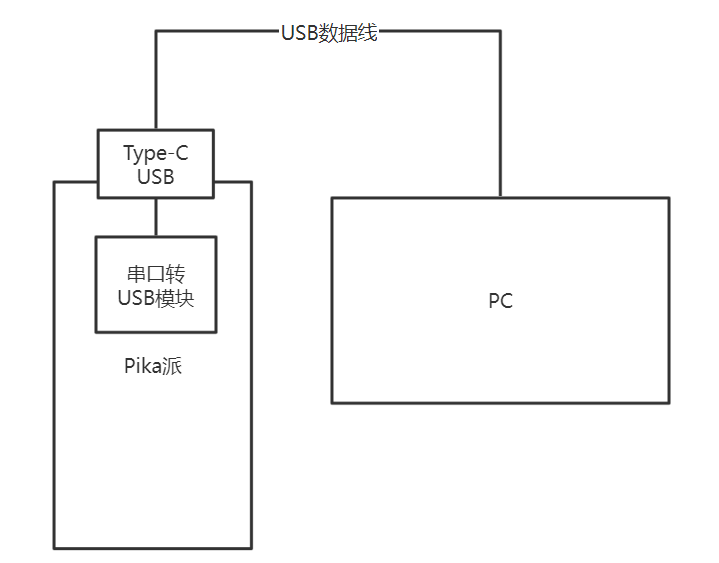https://gitee.com/Lyon1998/pikapython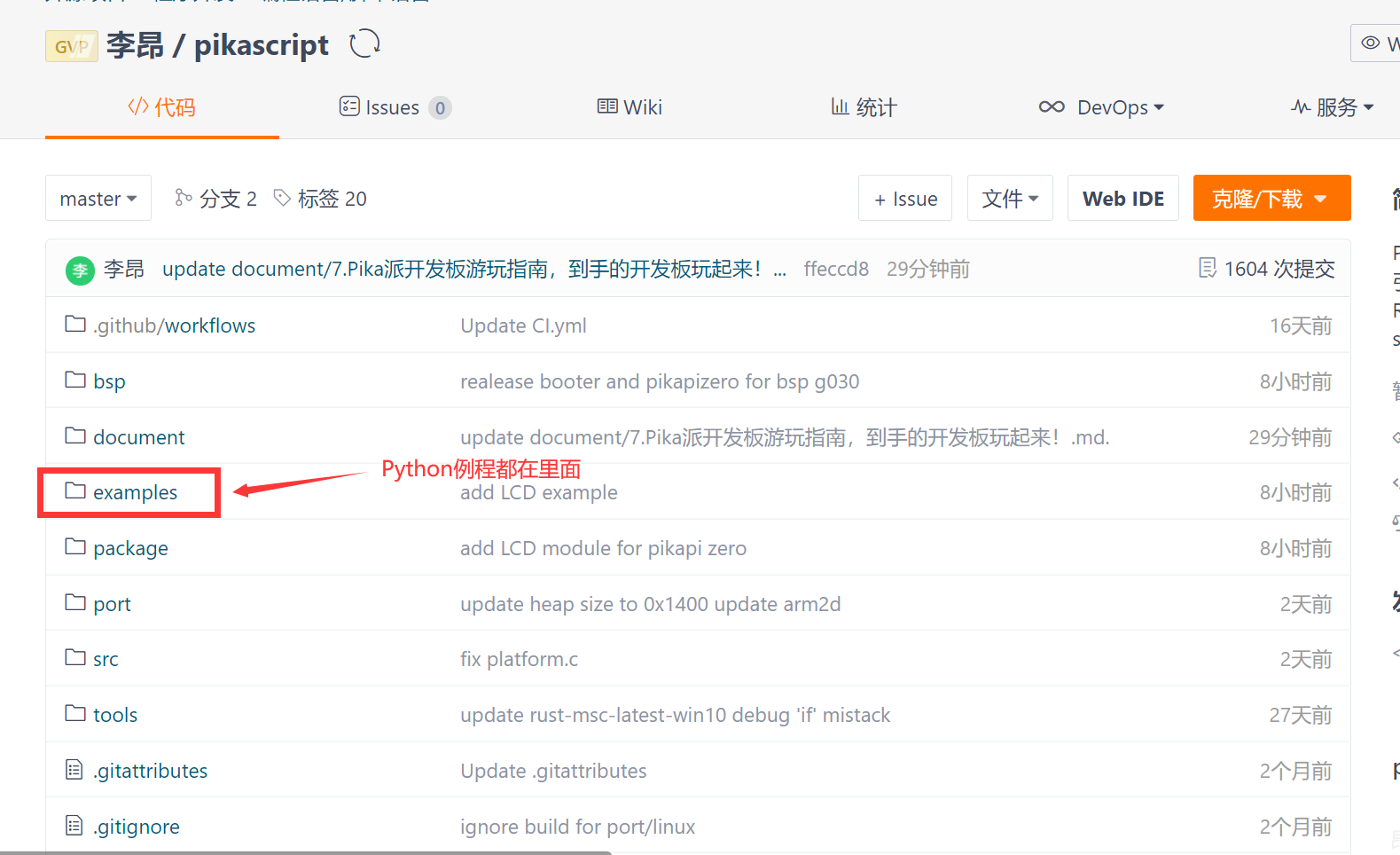Device 文件夹里面的 GPIO.py 就是 GPIO 的示例代码

```import PikaStdLib
import machine

mem = PikaStdLib.MemChecker()
io1 = machine.GPIO()
time = machine.Time()

io1.setPin('PA8')
io1.setMode('out')
io1.enable()
io1.low()

print('hello pikapython')
print('mem.max :')
mem.max()
print('mem.now :')
mem.now()

while True:
io1.low()
time.sleep_ms(500)
io1.high()
time.sleep_ms(500)

```

[注意]

1. python 文件开头应当包含 `import` 语句，才能触发 Python 文件下载功能。

2. 需要在脚本的末尾保留 2 个空行，确保识别到文件结尾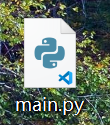## 3.2.5. GPIO的脚本里写了什么?

```import PikaStdLib
import machine
```

PikaStdLib 是 PikaPython 的标准库，里面有一些系统的功能，比如可以检查内存的占用。第四行里面，我们就新建了一个 mem 对象，这个对象的类是 PikaStdLib.MemChecker()。

```mem = PikaStdLib.MemChecker()
```

```print('hello pikapython')
print('mem.max :')
mem.max()
print('mem.now :')
mem.now()
```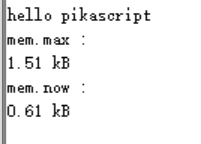time 对象是通过 machine 的 Time() 类新建的，可以提供基本的延时功能。

```time = machine.Time()
```

```time.sleep_ms(500)
```

io1 就是我们今天的主角了，这是一个 GPIO 对象，是用 machine .GPIO() 类新建的。

```io1 = machine.GPIO()
```

PA8 上连接了 Pika 派上面的一个 led 灯，只要控制 PA8 的电平，就可以控制灯的亮灭了。

```io1.setPin('PA8')
io1.setMode('out')
io1.enable()
io1.low()
```

```while True:
io1.low()
time.sleep_ms(500)
io1.high()
time.sleep_ms(500)
```

## 3.2.6. 其他的Python例程解读

```import PikaStdLib
import machine

time = machine.Time()

while True:
print('adc1 value:') # print out the value
print(val)
time.sleep_ms(500) # wait 0.5s

```

### 3.2.6.2. UART

```import PikaStdLib
import machine

time = machine.Time()
uart = machine.UART() #create a uart object
uart.setId(1) # set number of uart
uart.setBaudRate(115200) # set baudrate
uart.enable() #启动硬件

while True:
time.sleep_ms(500)
print(readBuff) # print out the char
```

### 3.2.6.3. PWM

```import PikaStdLib
import machine

time = machine.Time()
pwm = machine.PWM()
pwm.setPin('PA8') # setup the pin numbcer
pwm.setFrequency(2000) # setup the frequency
pwm.setDuty(0.5) # set the duty to 50%
pwm.enable()

while True:
time.sleep_ms(500)
pwm.setDuty(0.5)
time.sleep_ms(500)
pwm.setDuty(0.001) # set the duty to 0.1%
```

### 3.2.6.4. RGB

```import machine

import PikaStdLib

time = machine.Time()
pin = machine.GPIO()
pwm = machine.PWM()
uart = machine.UART()
rgb = machine.RGB() # create an RGB object
mem = PikaStdLib.MemChecker()

rgb.init() # init the object
rgb.enable() # launch the hardware

print('hello 2')
print('mem used max:')
mem.max()

while True:
print('flowing')
rgb.flow() # let RGB flow

```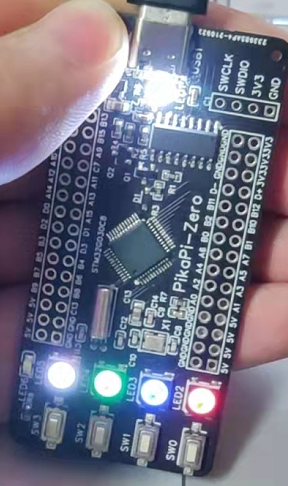### 3.2.6.5. LCD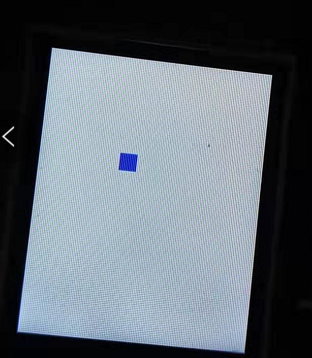```import PikaStdLib
import machine

lcd = machine.LCD()
lcd.init()
lcd.clear('white') # init LCD set background to white
mem = PikaStdLib.MemChecker()
key = machine.KEY() # new a KEY object
key.init()
time = machine.Time()
h = 10
w = 10
x = 10
y = 10 #Used to represent the height, width, and coordinates of small squares
x_last = x
y_last = y #Record the last location and use it for erasure
is_update = 0 #A flag variable that controls the refresh screen
print('mem used max:')
mem.max()
lcd.fill(x, y, w, h, 'blue') #Draw small blue squares
while True:
key_val = key.get() # Gets the value of the key
if key_val != -1:
x_last = x
y_last = y
is_update = 1 #Start the refresh
if key_val == 0:
x = x + 5 #Change the coordinates of small squares
if key_val == 1:
y = y - 5
if key_val == 2:
y = y + 5
if key_val == 3:
x = x - 5
if is_update: #Refresh the screen
is_update = 0
lcd.fill(x_last, y_last, w, h, 'white') #Erase off the previous position
lcd.fill(x, y, w, h, 'blue') #Draw the new location
```

## 3.2.7. 交互式运行

main.py 执行完毕后，就会进入交互式运行，因此只要取消 main.py 中的 `while True :`，使其能够执行完退出，就可以进入交互式运行。

• 强烈建议使用 pikaStudio 作为串口终端。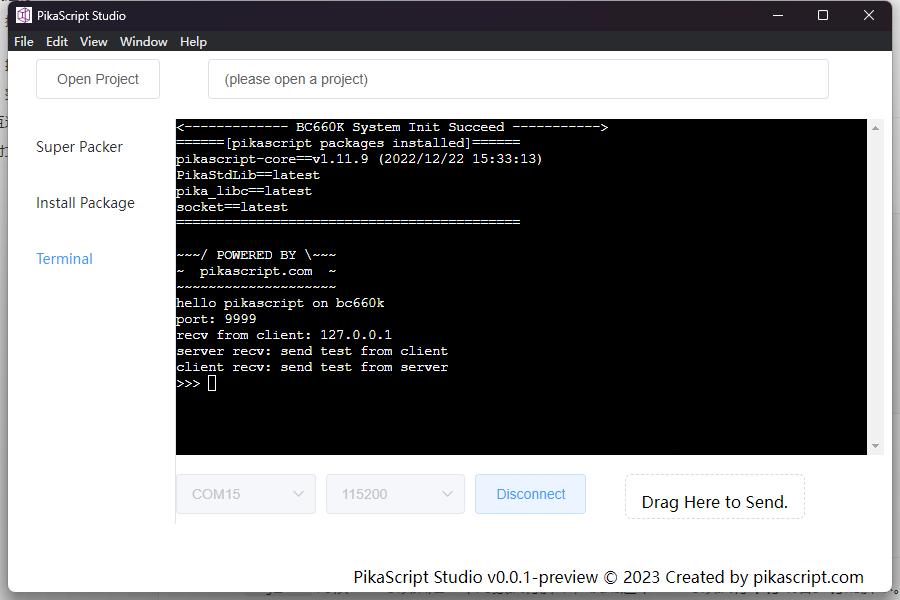[注意]

1. 固件版本需要不低于 `v1.3.2`

2. 如果使用 PuTTY 终端无法正常运行，请使用 XCOM。

3. 在终端中应全部使用英文输入法。

4. 缩进应使用 4 个空格，不要使用 TAB 键。

## 3.2.8. LCD 屏幕安装

1. 参考下图焊接长脚排母1. 插上屏幕，参考绿色小旗的方向，屏幕能亮就说明插的方向是对的，插反了不会亮1 模块找不到

2 工程编译报错，缺少文件：

## 3.2.10. 原理图

### 3.2.10.1. Lite 青春版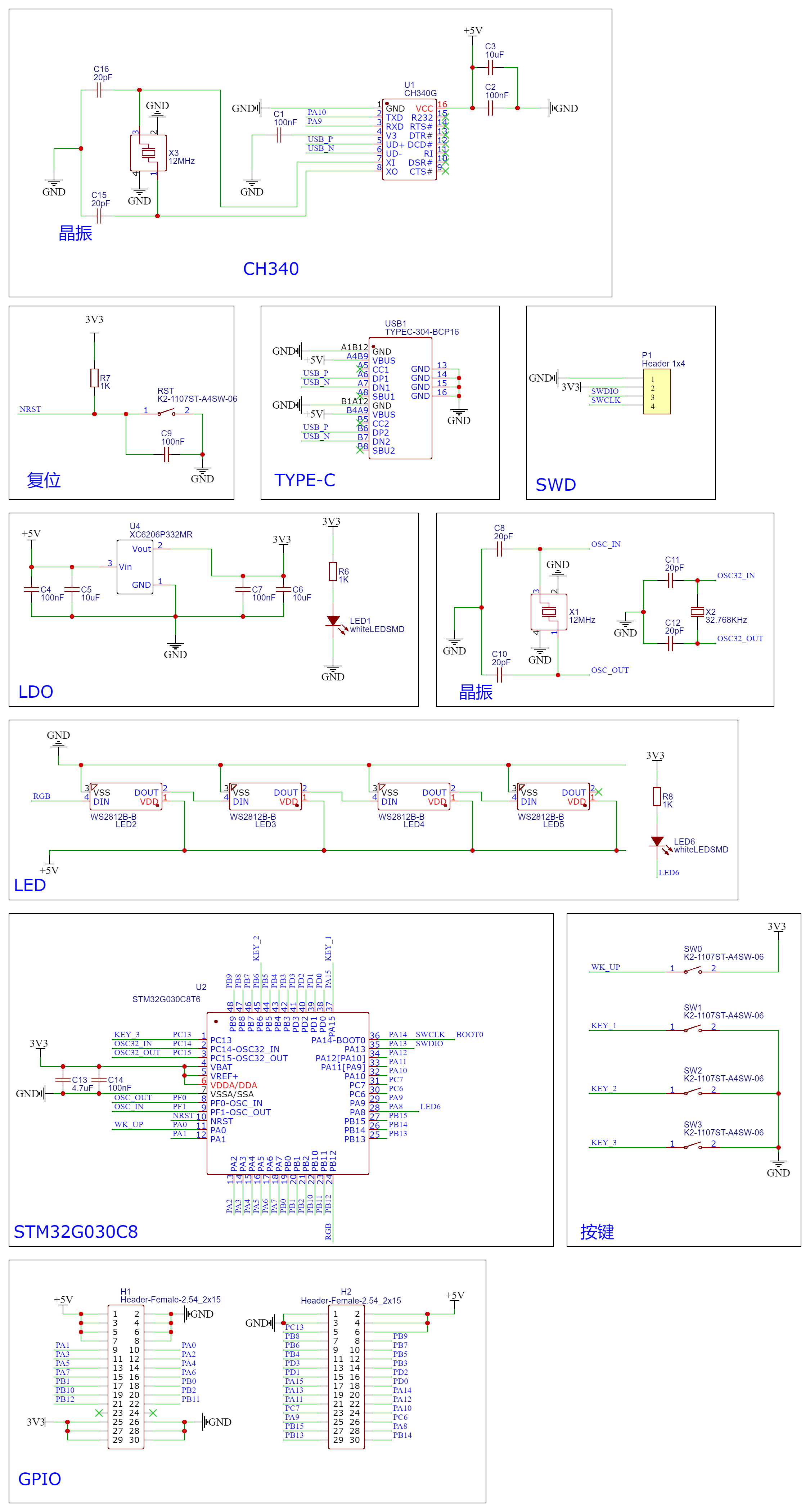### 3.2.10.2. Pro 专业版### 3.2.10.3. Plus 顶配版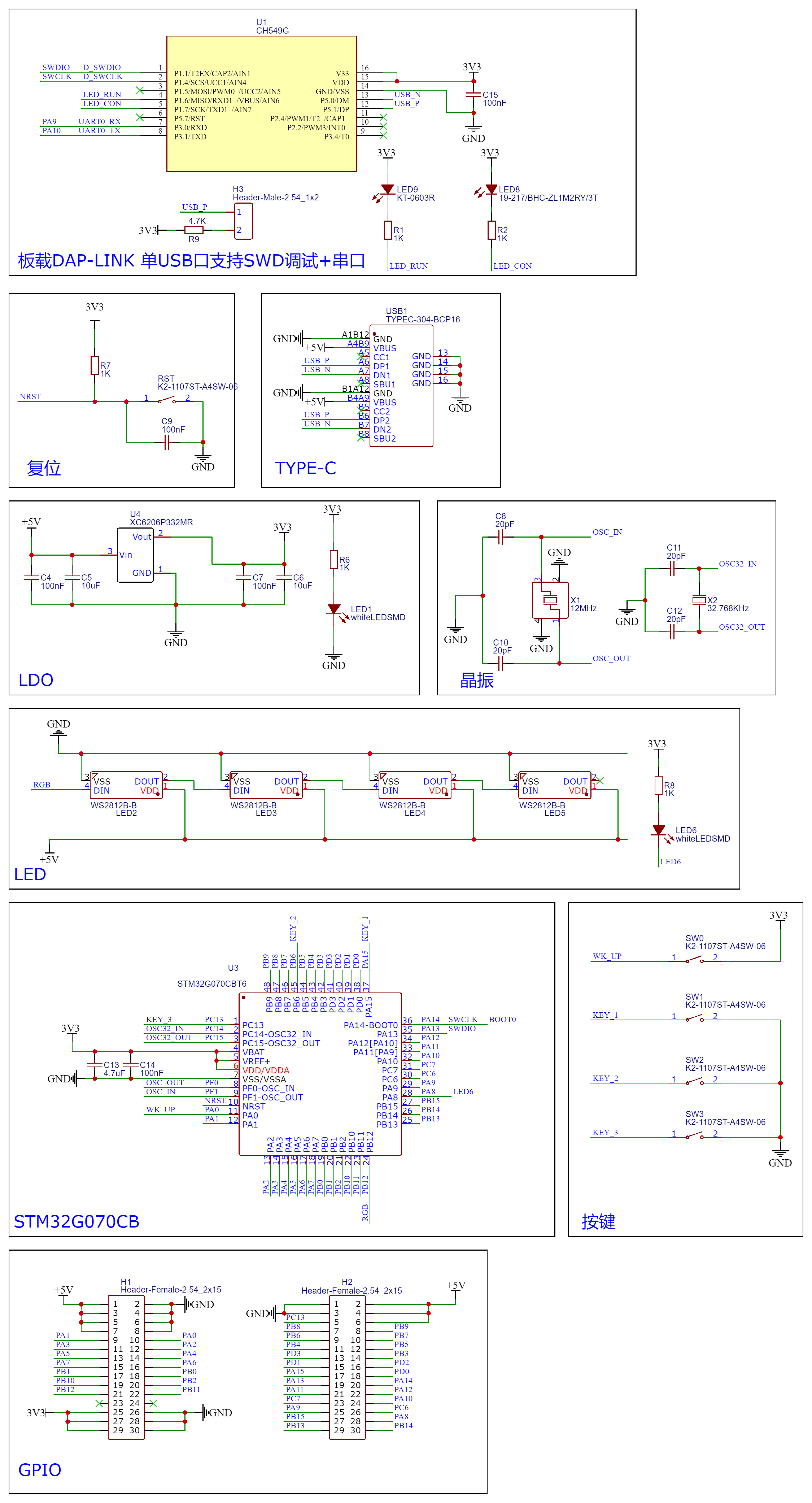### 3.2.10.4. LCD